# How to validate if input in input field has hexadecimal number only using express-validator ?

• Last Updated : 07 Apr, 2022

In HTML forms, we often required validation of different types. Validate existing email, validate password length, validate confirm password, validate to allow only integer inputs, these are some examples of validation. In a certain input field, only hexadecimal numbers are allowed. We can also validate these input fields to only accept full-width strings using express-validator middleware.

Hexadecimal uses sixteen as a base. Since most computers consist of elements that can only have two stable states, (such as ON or OFF), we use binary (base two) to represent the bits (binary digits) of a set of states in the computer. However, humans, who are more comfortable with number of bases that are somewhat bigger than two. Therefore, it was found to be more convenient to write numbers representing computer memory with fewer digits where each digit comes from a larger set (such as {0123456789ABCDEF} for “Hexadecimal”, which uses base-16).

Command to install express-validator:

`npm install express-validator`

Steps to use express-validator to implement the logic:

• Install express-validator middleware.
• Create a validator.js file to code all the validation logic.
• Validate input by validateInputField: check(input field name) and chain on validation isHexadecimal() with ‘.’
• Use the validation name(validateInputField) in the routes as a middleware as an array of validations.
• Destructure ‘validationResult’ function from express-validator to use it to find any errors.
• If error occurs redirect to the same page passing the error information.
• If error list is empty, give access to the user for the subsequent request.

Note: Here we use local or custom database to implement the logic, the same steps can be followed to implement the logic in a regular database like MongoDB or MySql.

Example: This example illustrates how to validate an input field to only allow hexadecimal numbers.

Filename – index.js

## javascript

 `const express = require(``'express'``)` `const bodyParser = require(``'body-parser'``)` `const {validationResult} = require(``'express-validator'``)` `const repo = require(``'./repository'``)` `const { validateIdentificationNumber } = require(``'./validator'``)` `const formTemplet = require(``'./form'``)`   `const app = express()` `const port = process.env.PORT || 3000`   `// The body-parser middleware to parse form data` `app.use(bodyParser.urlencoded({extended : ``true``}))`   `// Get route to display HTML form` `app.get(``'/'``, (req, res) => {` `  ``res.send(formTemplet({}))` `})`   `// Post route to handle form submission logic and ` `app.post(` `  ``'/info'``,` `  ``[validateIdentificationNumber],` `  ``async (req, res) => {` `    ``const errors = validationResult(req)`   `    ``if``(!errors.isEmpty()) {` `      ``return` `res.send(formTemplet({errors}))` `    ``}`   `    ``const {name, idn} = req.body`   `    ``// New record` `    ``await repo.create({` `      ``'Name'``:name,` `      ``'Identification Number'``:idn` `    ``})` `    ``res.send(``'Registered successfully..!!'``)` `})`   `// Server setup` `app.listen(port, () => {` `  ``console.log(`Server start on port \${port}`)` `})`

Filename – repository.js: This file contains all the logic to create a local database and interact with it.

## javascript

 `// Importing node.js file system module ` `const fs = require(``'fs'``)`   `class Repository {`   `  ``constructor(filename) {`   `    ``// Filename where data are going to store` `    ``if``(!filename) {` `      ``throw` `new` `Error(` `'Filename is required to create a datastore!'``)` `    ``}`   `    ``this``.filename = filename`   `    ``try` `{` `      ``fs.accessSync(``this``.filename)` `    ``} ``catch``(err) {`   `      ``// If file not exist it is created` `      ``// with empty array` `      ``fs.writeFileSync(``this``.filename, ``'[]'``)` `    ``}` `  ``}`   `  ``// Get all existing records` `  ``async getAll() {` `    ``return` `JSON.parse(` `      ``await fs.promises.readFile(``this``.filename, {` `        ``encoding : ``'utf8'` `      ``})` `    ``)` `  ``}` `  `  `  ``// Create new record` `  ``async create(attrs) {` `    ``// Fetch all existing records` `    ``const records = await ``this``.getAll()`   `    ``// All the existing records with new` `    ``// record push back to database` `    ``records.push(attrs)` `    ``await fs.promises.writeFile(` `      ``this``.filename,` `      ``JSON.stringify(records, ``null``, 2)   ` `    ``)` `    ``return` `attrs` `  ``}` `}`   `// The 'datastore.json' file created at runtime ` `// and all the information provided via signup form` `// store in this file in JSON format.` `module.exports = ``new` `Repository(``'datastore.json'``)`

Filename – form.js: This file contains logic to show the form.

## javascript

 `const getError = (errors, prop) => {` `  ``try` `{` `    ``return` `errors.mapped()[prop].msg` `  ``} ``catch` `(error) {` `    ``return` `''` `  ``} ` `}`   `module.exports = ({errors}) => {` `  ``return` ``` `` ``   `` `  ``` `  ``` ``   `` `  ``
` `    ``
` `      ``
` `        ``
` `          ``
` `            ``
` `              ``` `            ``
` `            ``` `          ``
` `          ``
` `            ``
` `              ``` `            ``
` `            ``` `            ``

` `              ``\${getError(errors, ``'idn'``)}` `            ``

` `          ``
` `          ``
` `            ``` `          ``
` `        ``` `      ``
` `    ``
` `  ``
` ``   `` `  ```` `}`

Filename – validator.js: This file contain all the validation logic(Logic to validate a input field to only allow hexadecimal numbers).

## javascript

 `const {check} = require(``'express-validator'``)` `const repo = require(``'./repository'``)` `module.exports = {` `  `  `  ``validateIdentificationNumber : check(``'idn'``)`   `    ``// To delete leading and trailing space` `    ``.trim()`   `    ``// Validate identification number to ` `    ``// accept only hexadecimal number` `    ``.isHexadecimal()`   `    ``// Custom message` `    ``.withMessage(``'Must be a hexadecimal number'``)   ` `}`

Filename – package.json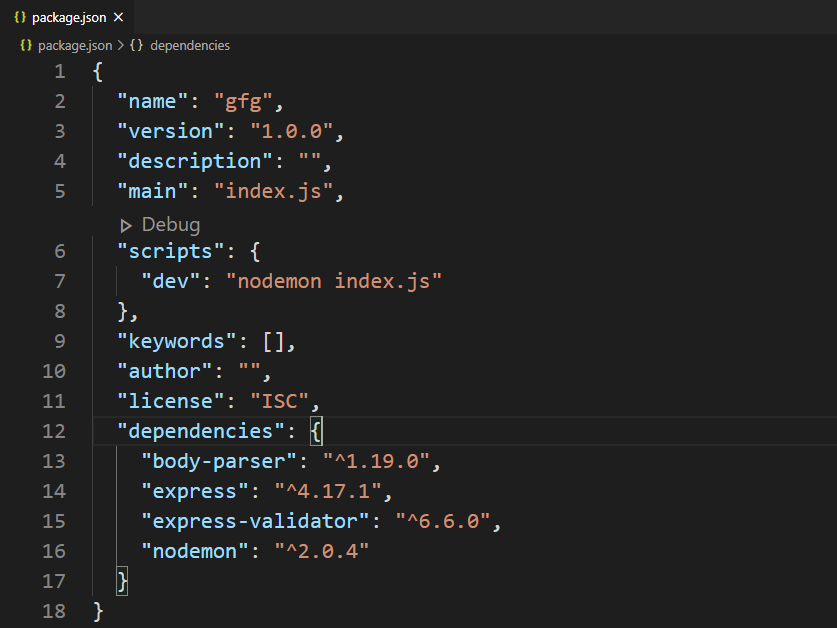package.json file

Database: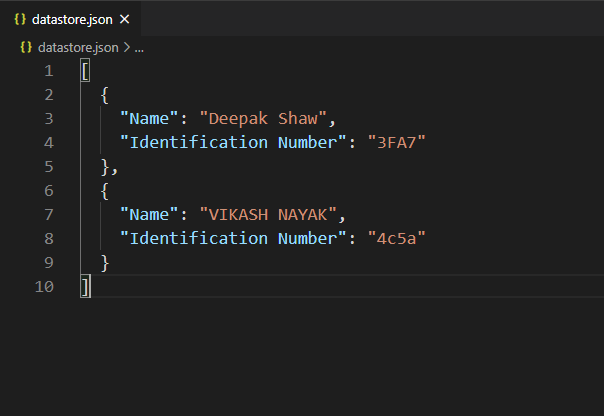Database

Output: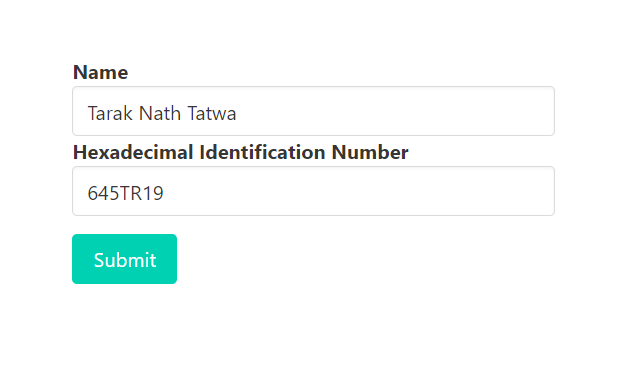Attempt to submit form data when identification number input field is not a valid hexadecimal number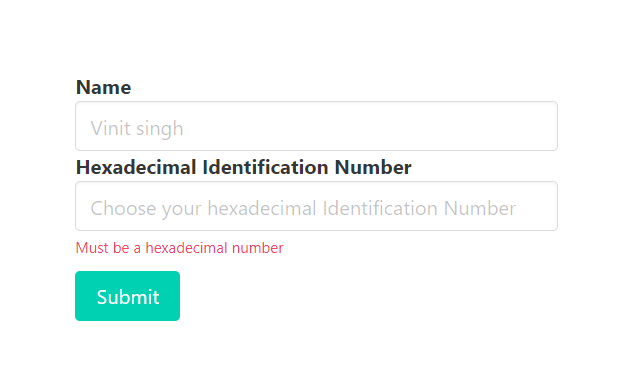Response when attempt to submit form data where identification number input field is  not a valid hexadecimal number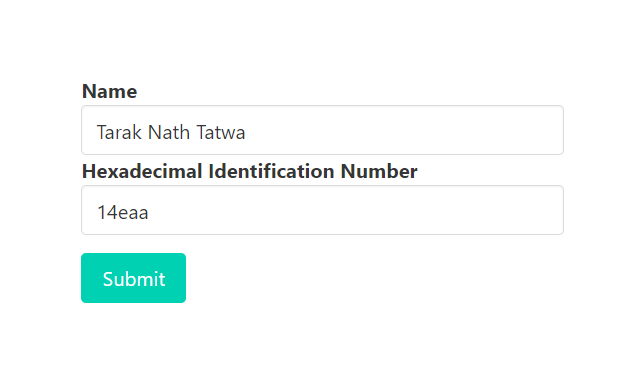Attempt to submit form data when identification number input field is  a valid hexadecimal numberResponse when attempt to submit form data where identification number input field is a valid hexadecimal number

Database after successful form submission: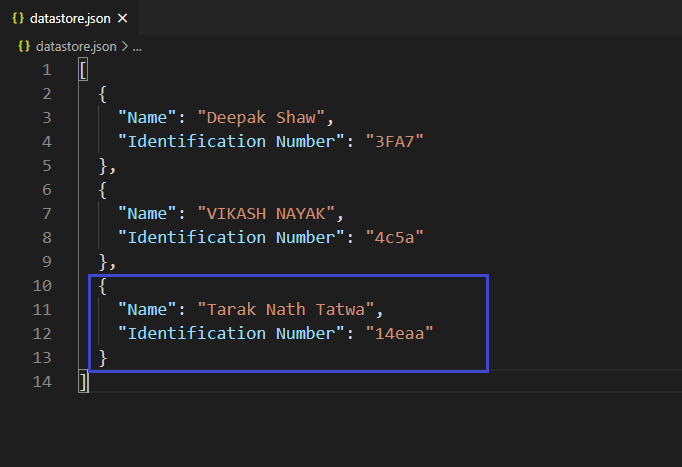Database after successful submission of form

Note: We have used some Bulma classes(CSS framework) in the form.js file to design the content.

My Personal Notes arrow_drop_up
Recommended Articles
Page :